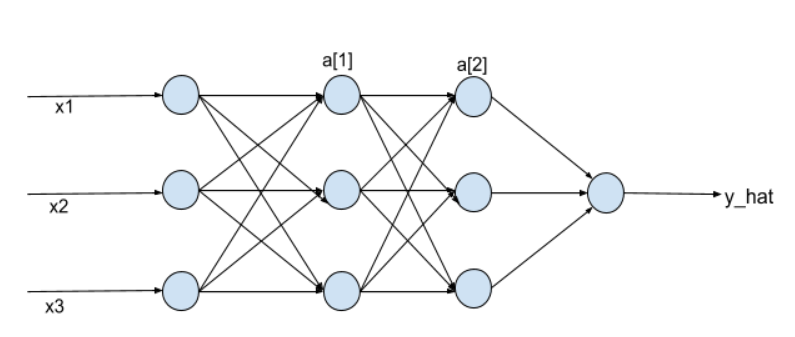# Why should we use Batch Normalization?

Why should we use Batch Normalization?

by (6.3k points)

Once the interviewer has asked you about the fundamentals of deep learning architectures, they would move on to the key topic of improving your deep learning model’s performance.

Batch Normalization is one of the techniques used for reducing the training time of our deep learning algorithm. Just like normalizing our input helps improve our logistic regression model, we can normalize the activations of the hidden layers in our deep learning model as well:We basically normalize a and a here. This means we normalize the inputs to the layer, and then apply the activation functions to the normalized inputs.

Here is an article that explains Batch Normalization and other techniques for improving Neural Networks: Neural Networks – Hyperparameter Tuning, Regularization & Optimization.

by (32.2k points)

Batch normalization is a technique for training very deep neural networks that standardizes the inputs to a layer for each mini-batch.

Usually, a dataset is fed into the network in the form of batches where the distribution of the data differs for every batch size. By doing this, there might be chances of vanishing gradient or exploding gradient when it tries to backpropagate. In order to combat these issues, we can use BN (with irreducible error) layer mostly on the inputs to the layer before the activation function in the previous layer and after fully connected layers.

Batch Normalisation has the following effects on the Neural Network:

1. Robust Training of the deeper layers of the network.
2. Better covariate-shift proof NN Architecture.
3. Has a slight regularisation effect.
4. Centred and Controlled values of Activation.
5. Tries to Prevent exploding/vanishing gradient.
6. Faster Training/Convergence to the minimum loss function